Test: Differential Amplifiers

# Test: Differential Amplifiers - Electronics and Communication Engineering (ECE)

Test Description

## 10 Questions MCQ Test Topicwise Question Bank for Electronics Engineering - Test: Differential Amplifiers

Test: Differential Amplifiers for Electronics and Communication Engineering (ECE) 2023 is part of Topicwise Question Bank for Electronics Engineering preparation. The Test: Differential Amplifiers questions and answers have been prepared according to the Electronics and Communication Engineering (ECE) exam syllabus.The Test: Differential Amplifiers MCQs are made for Electronics and Communication Engineering (ECE) 2023 Exam. Find important definitions, questions, notes, meanings, examples, exercises, MCQs and online tests for Test: Differential Amplifiers below.
Solutions of Test: Differential Amplifiers questions in English are available as part of our Topicwise Question Bank for Electronics Engineering for Electronics and Communication Engineering (ECE) & Test: Differential Amplifiers solutions in Hindi for Topicwise Question Bank for Electronics Engineering course. Download more important topics, notes, lectures and mock test series for Electronics and Communication Engineering (ECE) Exam by signing up for free. Attempt Test: Differential Amplifiers | 10 questions in 30 minutes | Mock test for Electronics and Communication Engineering (ECE) preparation | Free important questions MCQ to study Topicwise Question Bank for Electronics Engineering for Electronics and Communication Engineering (ECE) Exam | Download free PDF with solutions
 1 Crore+ students have signed up on EduRev. Have you?
Test: Differential Amplifiers - Question 1

### A resitive loaded and biased differential amplifier circuit is shown in figure. Neglecting the base currents and assuming matched transistors with VA = ∞,β = 100, w hat are the values of Rc and RE to meet the following specifications? Differential mode gain (double ended) = -500, Common mode rejection ratio = 500, Differential mode input resistance = 2 MQ.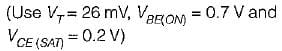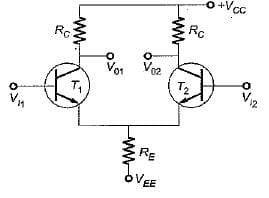Detailed Solution for Test: Differential Amplifiers - Question 1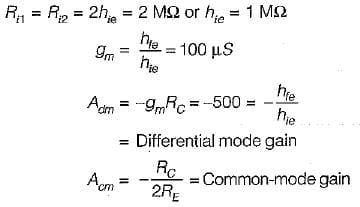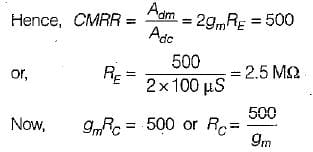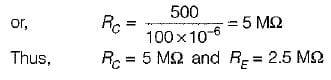Test: Differential Amplifiers - Question 2

### In the level shifter circuit shown in figure beiow, the internal drop across each diode VD = VBE2 = 0.7 V and hfe is very large. The value of R for V0 to become zero is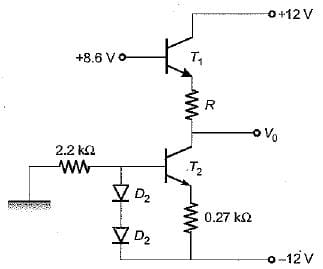Detailed Solution for Test: Differential Amplifiers - Question 2

The aiven circuit is redrawn as shown below.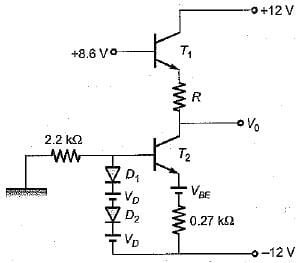Writing loop equation containing two diodes and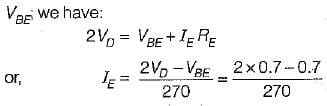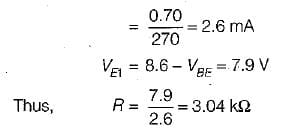Test: Differential Amplifiers - Question 3

### A differential amplifier, amplifies

Detailed Solution for Test: Differential Amplifiers - Question 3

The goal of designing differential amplifiers is to minimize the effect of common-mode input signal and amplify the difference of the voltages between the two input lines.

Test: Differential Amplifiers - Question 4

Most of linear ICs are based on the two transistor differential amplifier because of its

Test: Differential Amplifiers - Question 5

A differential amplifier has RL = 10 kΩ(equal values in both collectors), hie = 1 kΩ, Re = 50 kΩ, hte = 100. The common mode gain is given by

Detailed Solution for Test: Differential Amplifiers - Question 5

Common- mode gain,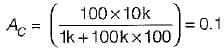Test: Differential Amplifiers - Question 6

In a single-stage differential amplifier, the output offset voltage is basically dependent on the mismatch of

Detailed Solution for Test: Differential Amplifiers - Question 6

In single stage differential amplifier, the output offset voltage is basically dependent on the mismatch of IB and β.

Test: Differential Amplifiers - Question 7

Assertion (A): It is not so easy to design d.c. amplifiers using transistors.
Reason (R): The values of hfe ,VBE and ICBO vary with temperature.

Detailed Solution for Test: Differential Amplifiers - Question 7

The values of hFE, VBE and ICB0 vary with temperature and due to the variation in any of these quantities the output voltage may change which may not be distinguished from a change in the input signal voltage, Due to this reason it is not so easy to design d.c. amplifiers using transistors. Hence, both assertion and reason are true and reason is the correct explanation of assertion.

Test: Differential Amplifiers - Question 8

Assertion (A): The ability of a differential amplifier to reject a differential mode signal is called its “figure of merit”.
Reason (R): The ideal value of figure of merit of a differential amplifier is infinite.

Detailed Solution for Test: Differential Amplifiers - Question 8

Assertion is not true because “figure of merit” (or CM MR) of a differential amplifier is to reject a common mode signal (vC).

Test: Differential Amplifiers - Question 9

A differential amplifier has (i) CMMR= 1000 and (ii) CMRR= 10000.
The first set of inputs is V1= 100 μV and V2, = -100μV.
The second set of inputs is V1= 1100 μV and V2 = 900 μV.
What is the percentage difference in output voltage obtained for the two sets of input voltages?

Detailed Solution for Test: Differential Amplifiers - Question 9

we know that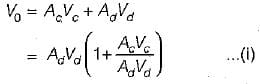Now,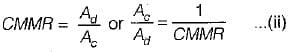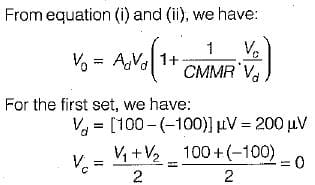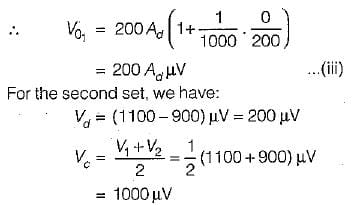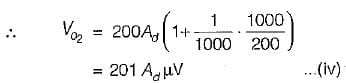From equation (iii) and (iv), the percentage difference in output voltage obtained for the two sets of input voltages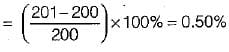Test: Differential Amplifiers - Question 10

The Q-point (Vc and IB) for the differential amplifier shown in figure below is given by: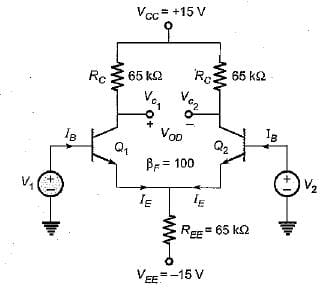Detailed Solution for Test: Differential Amplifiers - Question 10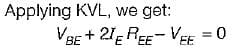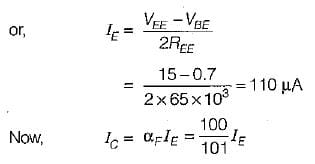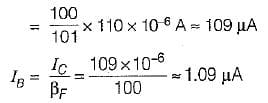Also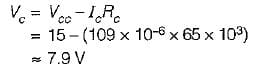Hence, both transistors of the differential amplifier are biased at a Q-point having lB = 1.09 μA and Vc=7.9V.

## Topicwise Question Bank for Electronics Engineering

193 tests
Information about Test: Differential Amplifiers Page
In this test you can find the Exam questions for Test: Differential Amplifiers solved & explained in the simplest way possible. Besides giving Questions and answers for Test: Differential Amplifiers, EduRev gives you an ample number of Online tests for practice

## Topicwise Question Bank for Electronics Engineering

193 tests(Scan QR code)